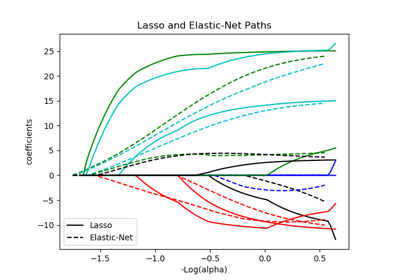# sklearn.linear_model.lasso_path¶

sklearn.linear_model.lasso_path(X, y, eps=0.001, n_alphas=100, alphas=None, precompute='auto', Xy=None, copy_X=True, coef_init=None, verbose=False, return_n_iter=False, positive=False, **params)[source]

Compute Lasso path with coordinate descent

The Lasso optimization function varies for mono and multi-outputs.

(1 / (2 * n_samples)) * ||y - Xw||^2_2 + alpha * ||w||_1


(1 / (2 * n_samples)) * ||Y - XW||^2_Fro + alpha * ||W||_21


Where:

||W||_21 = \sum_i \sqrt{\sum_j w_{ij}^2}


i.e. the sum of norm of each row.

Read more in the User Guide.

Parameters
X{array-like, sparse matrix}, shape (n_samples, n_features)

Training data. Pass directly as Fortran-contiguous data to avoid unnecessary memory duplication. If y is mono-output then X can be sparse.

yndarray, shape (n_samples,), or (n_samples, n_outputs)

Target values

epsfloat, optional

Length of the path. eps=1e-3 means that alpha_min / alpha_max = 1e-3

n_alphasint, optional

Number of alphas along the regularization path

alphasndarray, optional

List of alphas where to compute the models. If None alphas are set automatically

precomputeTrue | False | ‘auto’ | array-like

Whether to use a precomputed Gram matrix to speed up calculations. If set to 'auto' let us decide. The Gram matrix can also be passed as argument.

Xyarray-like, optional

Xy = np.dot(X.T, y) that can be precomputed. It is useful only when the Gram matrix is precomputed.

copy_Xboolean, optional, default True

If True, X will be copied; else, it may be overwritten.

coef_initarray, shape (n_features, ) | None

The initial values of the coefficients.

verbosebool or integer

Amount of verbosity.

return_n_iterbool

whether to return the number of iterations or not.

positivebool, default False

If set to True, forces coefficients to be positive. (Only allowed when y.ndim == 1).

**paramskwargs

keyword arguments passed to the coordinate descent solver.

Returns
alphasarray, shape (n_alphas,)

The alphas along the path where models are computed.

coefsarray, shape (n_features, n_alphas) or (n_outputs, n_features, n_alphas)

Coefficients along the path.

dual_gapsarray, shape (n_alphas,)

The dual gaps at the end of the optimization for each alpha.

n_itersarray-like, shape (n_alphas,)

The number of iterations taken by the coordinate descent optimizer to reach the specified tolerance for each alpha.

Notes

For an example, see examples/linear_model/plot_lasso_coordinate_descent_path.py.

To avoid unnecessary memory duplication the X argument of the fit method should be directly passed as a Fortran-contiguous numpy array.

Note that in certain cases, the Lars solver may be significantly faster to implement this functionality. In particular, linear interpolation can be used to retrieve model coefficients between the values output by lars_path

Examples

Comparing lasso_path and lars_path with interpolation:

>>> X = np.array([[1, 2, 3.1], [2.3, 5.4, 4.3]]).T
>>> y = np.array([1, 2, 3.1])
>>> # Use lasso_path to compute a coefficient path
>>> _, coef_path, _ = lasso_path(X, y, alphas=[5., 1., .5])
>>> print(coef_path)
[[0.         0.         0.46874778]
[0.2159048  0.4425765  0.23689075]]

>>> # Now use lars_path and 1D linear interpolation to compute the
>>> # same path
>>> from sklearn.linear_model import lars_path
>>> alphas, active, coef_path_lars = lars_path(X, y, method='lasso')
>>> from scipy import interpolate
>>> coef_path_continuous = interpolate.interp1d(alphas[::-1],
...                                             coef_path_lars[:, ::-1])
>>> print(coef_path_continuous([5., 1., .5]))
[[0.         0.         0.46915237]
[0.2159048  0.4425765  0.23668876]]


## Examples using sklearn.linear_model.lasso_path¶